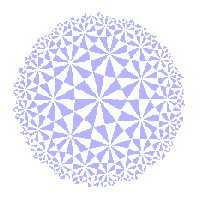# The Klein View of Geometry

The inversive group

 In the inversive geometry pages, we introduced inversive geometry. This is defined on the set C+ = Cu{∞}. We defined i-lines and inversion in i-lines. Here, we determine the group I(2) generated by such inversions. This is the inversive group. Its elements are inversive transformations. We know that each inversion preseves the size of angles, but reverses their sense. Thus I(2) has a subgroup I+(2) consisting of those which preserve the sense of angles. These are known as direct transformations. The others are indirect. An i-line is either a circle or an extended line (a euclidean line plus the point ∞). In the case of an extended line L, inversion with respect to L is simply reflection in the euclidean line, extended by mapping ∞ to ∞. By Theorem E1, E(2) is generated by the reflections. The extended versions generate a subgroup E(2) of I(2) which is isomorphic to E(2). In particular, I(2) contains extended versions of reflection in the real axis: r0(z)=z*, the translations : tc(z)=z+c, We can also extend elements of S(2) to C+ by defining s(∞)=∞ for each sεS(2) These extended versions form a group S(2) isomorphic to S(2). This is also a subgroup of I(2), as we now show. The result is of interest in its own right. The ∞ Theorem S(2) is the subgroup of I(2) consisting of elements which fix ∞. However, not all elements of I(2) belong to S(2). For example, ir, inversion in the circle |z|=r is given by ir(z)=r2/z*, when z ≠ 0, ∞ ir(0)=∞, ir(∞)=0. Note that the elements kr = iror0 have kr(z) = r2/z, z ≠ ∞, kr(∞) = ∞. They belong to I+(2) as composites of two inversions. We observe that the elements tc and kr of I+(2) can be written as Mobius transformations, i.e. in the form m(z) = (αz+β)/(γz+δ), where α, β, γ, δ are in C, with αδ - βγ ≠ 0. The set of all such transformations is the Mobius Group Theorem I1 I+(2) = M(2). This leads immediately to the corresponding theorem for the entire inversive group: Theorem I2 If tεI(2) is direct, then t(z) = m(z) for some m in M(2). If tεI(2) is indirect, then t(z) = m(z*) for some m in M(2). Proof If t is direct, we have the result by Theorem I1. If t is indirect, then tor0 is direct, so by Theorem I1, tor0 = mεM(2). Then t = mor0-1 = mor0, as required. With our knowledge of the groups, it is now possible to prove further results on the geometry, in particular, the Fundamental Theorem of Inversive Geometry.